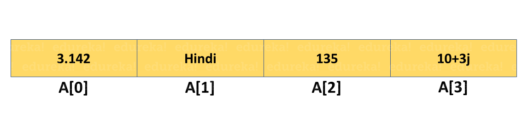# How to Find the Length of List in Python?

Last updated on Aug 09,2021 617.8K Views37 / 62 Blog from Python Fundamentals

The list in Python is a collection data-type that is ordered and changeable. A list can have duplicate entry as well. The Python len() method is used to find the length of any object. In this article, we will learn how to find the length of list in python in the following sequence:

## List in Python

A list in Python is implemented to store the sequence of various types of data. However, there are six data types in Python that are capable of storing the sequences but the most common and reliable type is a list.A list is defined as a collection of values or items of different types. The items in the list are separated with a comma (,) and enclosed with the square brackets [].

It is defined as follows:

```list1 = ['edureka', 'python', 2019];
list2 = [1, 2, 3, 4, 5 ];
list3 = ["a", "b", "c", "d"];```

If you want to learn Artificial Intelligence and Machine Learning in-depth, come to us and sign up for this Post Graduate Diploma AI and ML courses.

## How to Find the Length of List in Python?

There are two most commonly used and basic methods that are used to find the length of the list in Python:

• Len() Method
• Naive Method

## Len() Method

There is a built-in function called len() for getting the total number of items in a list, tuple, arrays, dictionary, etc. The len() method takes an argument where you may provide a list and it returns the length of the given list.

The len() method is one of the most used and convenient ways to find the length of list in Python. This is the most conventional technique adopted by all the programmers today.

Syntax:

`len(list)`

The List parameter is a list for which the number of elements are to be counted. It returns the number of elements in the list.

Example:

```ListName = ["Hello", "Edureka", 1, 2, 3]
print ("Number of items in the list = ", len(ListName))```

Output:5

## Naive Method

The len() method is the most commonly used method to find the length of the list in Python. But there is another basic method that provides the length of the list.

In the Naive method, one just runs a loop and increases the counter until the last element of the list to know its count. This is the most basic strategy that can be used in the absence of other efficient techniques.

Example:

```ListName = [ "Hello", "Edureka", 1,2,3 ]
print ("The list is : " + str(ListName))
counter = 0
for i in ListName:
counter = counter + 1
print ("Length of list using naive method is : " + str(counter))```

Output:

```The list is : ["Hello", "Edureka", 1,2,3]
Length of list using naive method is : 5```

This was all about finding the length of list in Python. The len() method is the most popular method. Whereas, you can also use the basic method of finding the length with the help of Naive Method.

With this, we have come to the end of our article. I hope you understood how to find the length of any list in Python.

To get in-depth knowledge of Python along with its various applications, you can enroll for live Python Certification Training with 24/7 support and lifetime access.

Got a question for us? Please mention it in the comments section of this “Length of List in Python” blog and we will get back to you as soon as possible.REGISTER FOR FREE WEBINARThank you for registering Join Edureka Meetup community for 100+ Free Webinars each month